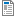National Curriculum

# Year 2/3 Maths Plans

Hamilton provide Mixed Year 2/3 weekly maths plans (below). We hope, in time, to develop maths blocks for this mixed year combination. Find out more about our plans to phase out these mixed age plans in favour of Year 2/3 maths blocks which we believe offer all the advantages of the weekly plans and more.

Supporting documents for set
• Week
• Title
1
+ Details
Number, place value and rounding

Year 2 objectives: Mark two-digit numbers on a landmarked line (labelled in tens); Compare numbers using the symbols < and >; Use ordinal numbers in context up to 10th and beyond; Use knowledge of order of numbers and properties of number (e.g. odd/even, multiples to describe/sort numbers); Solve logic problems; Round two-digit numbers to nearest multiple of ten. Year 3 objectives: Place 3-digit numbers on a line; Order and compare 3-digit numbers; Find a number between two 3-digit numbers; Understand place value in 3-digit numbers.Spring Week 1 Plan
•Spring Week 1 Image Resource
•ITP Number Grid
2
+ Details

Year 2: Add/subtract a single-digit to/from a 2-digit number by bridging multiples of ten using knowledge of pairs to ten and place value; Use place value or number facts to add subtract; Sort calculations as to how they can be worked out.  Year 3 Objectives: Add and subtract 1-digit numbers to and from 3-digit numbers; Add and subtract multiples of 10 and 100 to and from 3-digit numbers.Spring Week 2 Plan
3
+ Details

Year 2: Add/subtract two-digit numbers by adding/subtracting multiples of ten then ones (e.g. add 45 and 23 by adding 20, then 3) using a 1-100 grid, beaded line or landmarked line. Year 3: Add and subtract pairs of 2-digit numbers, including near multiples. Add three 2-digit numbers.

4
+ Details
2D shape and data

Year 2: Recognise common 2D shapes in different positions and orientations. Draw, sort and describe 2D shapes, discuss their properties including symmetry and right angles. Year 3: Recognise lines of symmetry, complete symmetrical drawings. Describe, name and sort 2D shapes. Identify whether angles are greater than or less than a right angle. Identify horizontal vertical, perpendicular and parallel lines.

5
+ Details
Place value and fractions

Year 2: Compare two 2-digit numbers and describe properties. Locate 2-digit numbers on a number line and find numbers in between. Round 2-digit numbers to the nearest 10. Find ½, 1/4s and 1/3 of amounts by sharing and using known number facts.  Year 3: Count in ¼s and ½s. Understand the concept of fractions. Find fractions with a total of 1. Find 1/4s and 1/8s, 1/3s and 1/6s of amounts. Includes bar model examples.

6
+ Details
Place value, multiplication and division

Year 2: Recognise multiples of 2s, 5s and 10s. Count in 2s and 5s from any number. Describe and continue patterns. Understand multiplication as repeated addition. Use multiplication sentences to describe an array and make links to division; Understand grouping as one model of division; Begin to understand remainders.  Year 3 Objectives: Understand place value in 3-digit numbers, including money; Multiply/divide numbers by 10 and 100; Understand that division is the inverse of multiplication.

7
+ Details

Year 2: Add 2-digit numbers using grid and Spider, and cross the tens barrier. Subtract 2-digit numbers using grid and Spider. Find the difference in the context of change.  Year 3: Add three-digit numbers using expanded addition (one ‘carry’). Count up to subtract two-digit numbers from three-digit numbers. Use addition to check subtraction.

8
+ Details
Time and data

Year 2: Measure using seconds/minutes and know the relationship between them. Know by heart the names and order of the days of the week and the names and order of the months of the year. Construct a block graph. Tell the time to the nearest ¼ hour then 5 minutes. Year 3: Time events in seconds. Collect data and record in scaled bar charts and pictograms. Read the time on a 12-hour digital clock and to the nearest 5 minutes on an analogue clock, convert time between analogue and digital. Find a time a number of minutes later.

9
+ Details

Year 2 Objectives: Sort calculations according to how they need to be worked out; use facts to add four or five small numbers. Sort word problems into whether addition or subtraction is needed. Year 3 Objectives: Add 3-digit numbers using expanded and compact addition; subtract by counting up to find the difference. Includes bar model examples.

10
+ Details
Mental multiplication and division

Year 2 Objectives: Understand how to read an array; Know that multiplication can be done in any order; Use beaded lines and grouping, then hops to work out division problems; Create own word problems involving division and multiplication; Sort division and multiplication word problems; Know that division is the inverse of multiplication. Year 3 Objectives: Use the 4 times table to learn the 8 times table; Recall 2, 3, 4, 5, 8 and 10 time tables; Use times tables to divide with remainders. Includes bar model examples.

11
+ Details